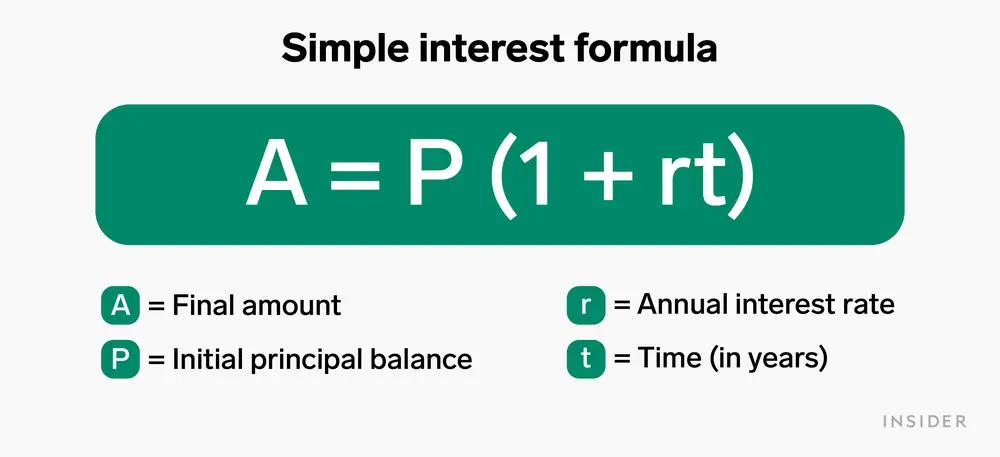# Simple Interest

The method of calculating the amount of interest charged on a sum of money at a given rate and a given period of time is known as simple interest. Unlike compound interest, the principal amount in the simple interest always remains the same. In the following article, we’ll discuss the principal, amount, interest rate, and time period. We’ll also learn about the formula to calculate simple interest.

## What is Simple Interest?

Simple interest is a quick and easy way to calculate money interest. With the simple interest method, interest is always applied to the initial principal amount, with the same interest rate for each time cycle. When we go to the bank and invest our money, then the bank gives interest on the amount. The interest that the bank provides, can be of many types, and one of them is the simple interest. Let us first understand the concept of a loan.

A particular amount that a person borrows from a bank or some financial authority for some needs is known as a loan. Some of the examples that come under the loan are home loans, educational loans, car loans, personal loans, etc. The amount of the loan is to be returned to the authority after a mentioned period, with an extra amount, which is called the interest on the original amount of the loan.

## Simple Interest Formula

The following factors are used to calculate the simple interest on any amount:

Principal(P), which is the original amount; Rate of Interest(R) in % per annum, is the interest rate that is considered while calculating the amount and lastly, time period(T), which is the duration provided for the amount to be returned.

• Principal(P)- The principal is the amount that is borrowed from the financial authority or invested in the beginning. The symbol used to denote is P.
• Rate(R)- It is the rate of interest at which the principal is given or borrowed for a certain duration. The rate is expressed in percentage and is denoted by R.
• Time(T)- Time represents the duration for which the principal amount is given or borrowed. The symbol that is used to denote time is T.
• Amount(A)- After borrowing the loan, the person has to return that amount as well as the mentioned interest. The total returned amount including the interest is known as the amount, which is denoted by A.

Therefore, the formula required to calculate the simple interest is,

S.I.= P×R×T

And, the formula for calculating the total amount to be returned is,

Amount = Principal + Simple Interest

A = P + S.I.

A = P + PRT

A = P (1 + RT)

### Compound Interest Formula

The interest that is paid on both the principal amount and the simple interest, compounded at regular time intervals is known as the compound interest. The compound interest formula is expressed as CI = P (1 + r/n)nt – P, given that P is the initial amount, r is the rate of interest at which the principal is given, n denotes the number of times the interest is compounded annually, and t implies the total duration taken.

### Difference Between Simple Interest and Compound Interest

Let us discuss some differences between the simple interest and the compound interest for a better understanding of the concept.

• Simple interest is calculated on the initial principal amount, whereas compound interest is calculated on the total sum of the principal and interest.
• Simple interest remains the same for every year, it doesn’t change, whereas compound interest is different for every time duration since it is calculated on the accumulated amount, and not just the principal.

To learn about the simple and the compound interests in a better and more fun way, visit Cuemath.